# The SEQDESIGN Procedure

## Getting Started: SEQDESIGN Procedure

This section illustrates a clinical study design that uses a two-sided O’Brien-Fleming design (O’Brien and Fleming 1979) to stop the trial early for ethical concerns about possible harm or for unexpectedly strong efficacy of the new drug.

Suppose that a pharmaceutical company is conducting a clinical trial to test the efficacy of a new cholesterol-lowering drug. The primary focus is low-density lipoprotein (LDL), the so-called bad cholesterol, which is a risk factor for coronary heart disease. LDL is measured in mg/dl, milligrams per deciliter of blood.

The trial consists of two groups of equally allocated patients with elevated LDL levels: an experimental group given the new drug and a placebo control group. Suppose the changes in LDL level after the treatment for individuals in the experimental and control groups are normally distributed with means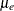and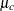, respectively, and have a common variance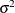. Then the null hypothesis of no effect for the new drug is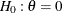, where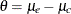.

For a fixed-sample design with a total sample size N, the MLE for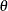is computed as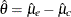, where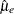and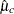are the sample means of the decreases in LDL level in the experimental and control groups, respectively.

Following the derivation in the section Test for the Difference between Two Normal Means, the statistic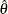has a normal distribution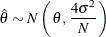Thus, under the null hypothesis, the standardized statistic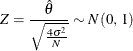The Z statistic can be used to test the null hypothesis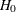. If the varianceis unknown, the sample variance can be used to compute the test statistic if it is assumed that the sample variance is computed from a large sample such that the Z statistic has an approximately standard normal distribution.

With a Type I error probability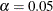, the critical values for the Z statistic are given by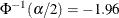and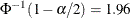, where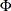is the cumulative standard normal distribution function. At the end of study, if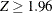, the null hypothesis is rejected for harmful drug effect, and if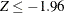, the null hypothesis is rejected for efficacy of the new drug. Otherwise, the null hypothesis is not rejected and the drug effect is not significant.

Also suppose that for the trial, the alternative reference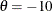is the clinically meaningful difference that the trial should detect with a high probability (power). Further suppose that a good estimate of the standard deviation for the changes in LDL level is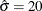. The following statements invoke the SEQDESIGN procedure and request a four-stage O’Brien-Fleming design for standardized normal test statistics:

ods graphics on;
proc seqdesign altref=-10
plots=boundary(hscale=samplesize)
;
TwoSidedOBrienFleming: design nstages=4
method=obf
;
samplesize model=twosamplemean(stddev=20);
ods output Boundary=Bnd_LDL;
run;


The ALTREF= option specifies the alternative reference, and the actual maximum information is derived in the SEQDESIGN procedure. With ODS Graphics enabled, the PLOTS=BOUNDARY option displays a boundary plot with the rejection and acceptance regions.

In the DESIGN statement, the label TwoSidedOBrienFleming identifies the design in the output tables. By default (or equivalently if you specify ALT=TWOSIDED and STOP=REJECT in the DESIGN statement), the design has a two-sided alternative hypothesis in which early stopping in the interim stages occurs to reject the null hypothesis. That is, at each interim stage, the trial either is stopped to reject the null hypothesis or continues to the next stage.

The NSTAGES=4 option in the DESIGN statement specifies the total number of stages in the group sequential trial, including three interim stages and a final stage. In the SEQDESIGN procedure, the null hypothesis for the design is. By default (or equivalently if you specify ALPHA=0.05 and BETA=0.10 in the DESIGN statement), the design has a Type I error probability, and a Type II error probability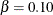; the latter corresponds to a power of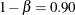at the alternative reference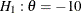.

For a two-sided design with early stopping to reject the null hypothesis, there are two boundaries for the design: an upperboundary that consists of upper rejection critical values and a lowerboundary that consists of lower rejection critical values. Each boundary is a set of critical values, one from each stage. With the METHOD=OBF option in the DESIGN statement, the O’Brien-Fleming method is used for the two boundaries for the design; see Figure 101.7.

A property of the boundaries constructed with the O’Brien-Fleming design is that the null hypothesis is more difficult to reject in the early stages than in the later stages. That is, the trial is rejected in the early stages only with overwhelming evidence, because in these stages there might not be a sufficient number of responses for a reliable estimate of the treatment effect.

The SAMPLESIZE statement with the MODEL=TWOSAMPLEMEAN option uses the derived maximum information to compute required sample sizes for a two-sample test for mean difference. The ODS OUTPUT statement with the BOUNDARY=BND_LDL option creates an output data set named BND_LDL which contains the resulting boundary information.

In a clinical trial, the amount of information about an unknown parameter available from the data can be measured by the Fisher information. For a maximum likelihood statistic, the information level is the inverse of its variance. See the section Maximum Likelihood Estimator for a detailed description of Fisher information. At each stage of the trial, data are collected and analyzed with a statistical procedure, and a test statistic and its corresponding information level are computed.

In this example, you can use the REG procedure to compute the maximum likelihood estimatefor the drug effect and the corresponding standard error for. At stage 1, you can use the SEQTEST procedure to compare the test statistic with adjusted boundaries derived from the boundary information stored in the BOUND_LDL data set. At each subsequent stage, you can use the SEQTEST procedure to compare the test statistic with adjusted boundaries derived from the boundary information stored in the test information table created by the SEQTEST procedure at the previous stage. The test information tables are structured for input to the SEQTEST procedure.

At each interim stage, the trial will either be stopped to reject the null hypothesis or continue to the next stage. At the final stage, the null hypothesis is either rejected or accepted.

By default (or equivalently if you specify INFO=EQUAL in the DESIGN statement), the SEQDESIGN procedure derives boundary values with equally spaced information levels for all stages—that is, the same information increment between successive stages. The "Design Information," "Method Information," and "Boundary Information" tables are displayed by default, as shown in Figure 101.4, Figure 101.5, and Figure 101.6, respectively.

The "Design Information" table in Figure 101.4 displays design specifications and four derived statistics: the actual maximum information, the maximum information, the average sample number under the null hypothesis (Null Ref ASN), and the average sample number under the alternative hypothesis (Alt Ref ASN). Except for the actual maximum information, each statistic is expressed as a percentage of the identical statistic for the corresponding fixed-sample information. The average sample number is the expected sample size (for nonsurvival data) or expected number of events (for survival data). Note that for a symmetric two-sided design, the ALTREF=–10 option implies a lower alternative reference of –10 and an upper alternative reference of 10.

The SEQDESIGN Procedure
Design: TwoSidedOBrienFleming

Statistic Distribution Normal
Boundary Scale Standardized Z
Alternative Hypothesis Two-Sided
Early Stop Reject Null
Method O'Brien-Fleming
Boundary Key Both
Alternative Reference -10
Number of Stages 4
Alpha 0.05
Beta 0.1
Power 0.9
Max Information (Percent of Fixed Sample) 102.2163
Max Information 0.107403
Null Ref ASN (Percent of Fixed Sample) 101.5728
Alt Ref ASN (Percent of Fixed Sample) 76.7397

The maximum information is the information level at the final stage of the group sequential trial. The Max Information (Percent Fixed-Sample) is the maximum information for the sequential design expressed as a percentage of the information for the corresponding fixed-sample design. In Figure 101.4, the Max Information (Percent Fixed-Sample) is 102.22%, which means that the information needed for the group sequential trial is 2.22% more than that of the corresponding fixed-sample design if the trial does not stop at any interim stage.

The Null Ref ASN (Percent Fixed-Sample) is the average sample number (expected sample size) required under the null hypothesis for the group sequential design expressed as a percentage of the sample size for the corresponding fixed-sample design. In Figure 101.4, the Null Ref ASN is 101.57%, which means that the expected sample size for the group sequential trial is 1.57% greater than the corresponding fixed-sample size.

Similarly, the Alt Ref ASN (Percent Fixed-Sample) is the average sample number (expected sample size) required under the alternative hypothesis for the group sequential design expressed as a percentage of the sample size for the corresponding fixed-sample design. In Figure 101.4, the Alt Ref ASN is 76.74%, which means that the expected sample size for the group sequential trial is 76.74% of the corresponding fixed-sample size. That is, if the alternative hypothesis is true, then on average, only 76.74% of the fixed-sample size is needed for the group sequential trial.

In this example, the O’Brien-Fleming design requires only a slight increase in sample size if the trial proceeds to the final stage. On the other hand, if the alternative hypothesis is correct, this design provides a substantial saving in sample size on average.

The "Method Information" table in Figure 101.5 displays the computed Type I and Type II error probabilitiesand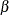, and the derived drift parameter for the design. For a two-sided test with early stopping to reject the null hypothesis, both lower and upperboundaries are created. With the specified ALTREF= option, the alternative references are also included.

With the zero null reference, the drift parameter is the standardized alternative reference at the final stage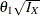, where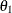is the alternative reference and
is the maximum information. See the section Specified and Derived Parameters for a detailed description of the drift parameter. The drift parameters for the design are derived in the SEQDESIGN procedure even if the alternative reference is not specified or derived in the procedure.

Figure 101.5: Method Information

Method Information
Boundary Method Alpha Beta Unified Family Alternative
Reference
Drift
Rho Tau C
Upper Alpha O'Brien-Fleming 0.02500 0.10000 0.5 0 2.02429 10 3.277238
Lower Alpha O'Brien-Fleming 0.02500 0.10000 0.5 0 2.02429 -10 -3.27724

The O’Brien-Fleming method belongs to the unified family of designs, which is parameterized by two parameters,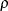and, as implemented in the SEQDESIGN procedure. See Table 101.3 for parameter values of commonly used methods in the unified family. The "Method Information" table in Figure 101.5 displays the values of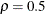and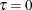, which are the parameters for the O’Brien-Fleming method. The table also displays the derived parameter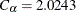, which is used in the construction of symmetric lower and upperboundaries; see the section Unified Family Methods.

The "Boundary Information" table in Figure 101.6 displays the information level, including the proportion, actual level, and corresponding sample size (N) at each stage. The table also displays the lower and upper alternative references, and the lower and upper boundary values at each stage.

Figure 101.6: Boundary Information

Boundary Information (Standardized Z Scale)
Null Reference = 0
_Stage_   Alternative Boundary Values
Information Level Reference Lower Upper
Proportion Actual N Lower Upper Alpha Alpha
1 0.2500 0.026851 42.96116 -1.63862 1.63862 -4.04859 4.04859
2 0.5000 0.053701 85.92233 -2.31736 2.31736 -2.86278 2.86278
3 0.7500 0.080552 128.8835 -2.83817 2.83817 -2.33745 2.33745
4 1.0000 0.107403 171.8447 -3.27724 3.27724 -2.02429 2.02429

The information proportion is the proportion of maximum information available at each stage and N is the corresponding sample size. By default (or equivalently if you specify BOUNDARYSCALE=STDZ), the procedure displays boundary values with the standardized Z scale in the boundary information table and the boundary plot. The alternative reference on the standardized Z scale at stage k is given by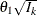, whereis the alternative reference and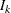is the information available at stage k,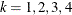. These standardized alternative references for the design are derived in the SEQDESIGN procedure even if the alternative reference is not specified or derived in the procedure.

In this example, a standardized Z statistic is computed by standardizing the parameter estimate of the effect in LDL level. A lower Z test statistic indicates a beneficial effect. Consequently, at each interim stage, if the standardized Z test statistic is less than or equal to the corresponding lowerboundary value, the hypothesisis rejected for efficacy. If the test statistic is greater than or equal to the corresponding upperboundary value, the hypothesisis rejected for harmful effect. Otherwise, the process continues to the next stage. At the final stage (stage 4), the hypothesisis rejected for efficacy if the Z statistic is less than or equal to the corresponding lowerboundary value –2.0243, and the hypothesisis rejected for harmful effect if the Z statistic is greater than or equal to the corresponding upperboundary value 2.0243. Otherwise, the hypothesis of no significant difference is accepted.

Note that in a typical trial, the actual information levels do not match the information levels specified in the design. The SEQTEST procedure modifies the boundary values stored in the BOUND_LDL data set to adjust for these new information levels.

With ODS Graphics enabled, a detailed boundary plot with the rejection and acceptance regions is displayed, as shown in Figure 101.7. This plot displays the boundary values in the "Boundary Information" table in Figure 101.6. The stages are indicated by vertical lines with accompanying stage numbers. The horizontal axis indicates the sample sizes for the stages. Note that comparing with a fixed-sample design, only a small increase in sample size is needed for the O’Brien-Fleming design, as shown in Figure 101.7.

If a test statistic at an interim stage is in the rejection region (shaded area), the trial stops and the null hypothesis is rejected. If the statistic is not in any rejection region, the trial continues to the next stage.

Figure 101.7: Boundary Plot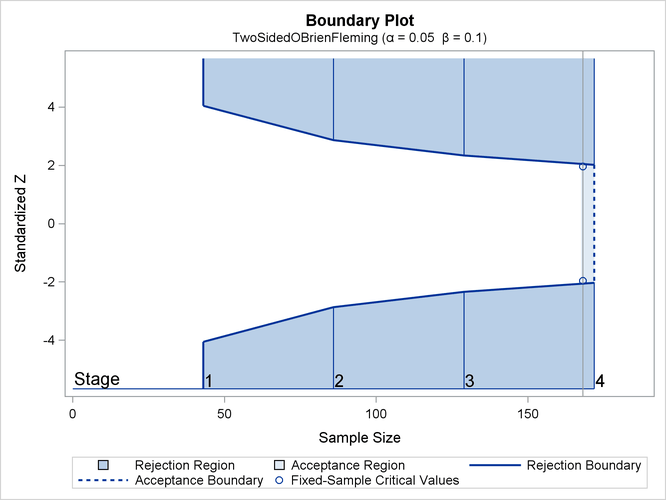The boundary plot also displays critical values for the corresponding fixed-sample design. The symbol "" identifies the fixed-sample critical values of –1.96 and 1.96, and the accompanying vertical line indicates the required sample size for the fixed-sample design at the horizontal axis. Note that the boundary values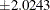at the final stage are close to the fixed-sample critical values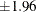.

When you specify the SAMPLESIZE statement, the maximum information (either explicitly specified or derived in the SEQDESIGN procedure) is used to compute the required sample sizes for the study. The MODEL=TWOSAMPLEMEAN(STDDEV=20) option specifies the test for the difference between two normal means. See the section Test for the Difference between Two Normal Means for a detailed derivation of these required sample sizes.

The "Sample Size Summary" table in Figure 101.8 displays the parameters for the sample size computation and the resulting maximum and expected sample sizes.

Figure 101.8: Sample Size Summary

Sample Size Summary
Test Two-Sample Means
Mean Difference -10
Standard Deviation 20
Max Sample Size 171.8447
Expected Sample Size (Null Ref) 170.7627
Expected Sample Size (Alt Ref) 129.0137

The "Sample Sizes (N)" table in Figure 101.9 displays the required sample sizes at each stage for the trial, in both fractional and integer numbers. The derived fractional sample sizes are displayed under the heading "Fractional N." These sample sizes are rounded up to integers under the heading "Ceiling N." By default (or equivalently if you specify WEIGHT=1 in the MODEL=TWOSAMPLEMEAN option), the sample sizes for the two groups are equal for the two-sample test.

Figure 101.9: Derived Sample Sizes

Sample Sizes (N)
Two-Sample Z Test for Mean Difference
_Stage_ Fractional N Ceiling N
N N(Grp 1) N(Grp 2) Information N N(Grp 1) N(Grp 2) Information
1 42.96 21.48 21.48 0.0269 44 22 22 0.0275
2 85.92 42.96 42.96 0.0537 86 43 43 0.0538
3 128.88 64.44 64.44 0.0806 130 65 65 0.0812
4 171.84 85.92 85.92 0.1074 172 86 86 0.1075

In practice, integer sample sizes are used in the trial, and the resulting information levels increase slightly. Thus, 22, 43, 65, and 86 individuals are needed in each of the two groups for the four stages, respectively.

You can also create an adjusted design that corresponds to these integer-valued sample sizes at the stages by specifying the CEILADJDESIGN=INCLUDE option in the SAMPLESIZE statement.